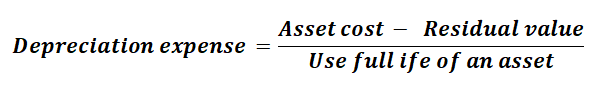Depreciation is an accounting method used to distribute the cost of a tangible or physical asset throughout its use. This method helps businesses understand how much of an asset’s value has been used. It also helps realize the revenue the asset generates while recording a fraction of the cost of the asset as expenses, every year.

This fraction of expense is called depreciation expense. There are different methods to calculate depreciation expenses. They are the Straight-Line method, the Declining balance method, the Double Declining Balance method, Sum-of-the-Year’s-Digits, and Units of production method.

Depreciation expense is calculated based on the nature of the asset. Some assets depreciate steadily, therefore the depreciation expense is equal throughout the lifespan of the asset. Others lose most of their value in the initial years causing the depreciation expense to decline over the years.

The Formula: